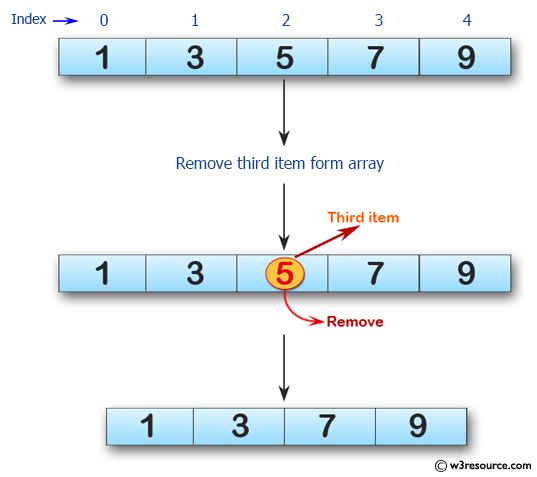﻿ Python: Remove a specified item using the index from an array - w3resource# Python: Remove a specified item using the index from an array

## Python: Array Exercise-11 with Solution

Write a Python program to remove a specified item using the index from an array.

Pictorial Presentation:Sample Solution:

Python Code :

``````from array import *
array_num = array('i', [1, 3, 5, 7, 9])
print("Original array: "+str(array_num))
print("Remove the third item form the array:")
array_num.pop(2)
print("New array: "+str(array_num))
``````

Sample Output:

```Original array: array('i', [1, 3, 5, 7, 9])
Remove the third item form the array:
New array: array('i', [1, 3, 7, 9])
```

Python Code Editor:

What is the difficulty level of this exercise?

Test your Python skills with w3resource's quiz

﻿

## Python: Tips of the Day

Python: Membership Testing in a Collection

```>>> a = ('one', 'two', 'three', 'four', 'five')
>>> if 'one' in a:
...     print('The tuple contains one.')
...
The tuple contains one.
>>> b = {0: 'zero', 1: 'one', 2: 'two', 3: 'three'}
>>> if 2 in b.keys():
...     print('The dict has the key of 2.')
...
The dict has the key of 2.
```